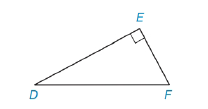Chapter 5.4, Problem 7E### Elementary Geometry for College St...

6th Edition
Daniel C. Alexander + 1 other
ISBN: 9781285195698

#### Solutions

Chapter
Section### Elementary Geometry for College St...

6th Edition
Daniel C. Alexander + 1 other
ISBN: 9781285195698
Textbook Problem
1 views

# Find the length of D F ¯ if:a) D E = 8   and E F = 6 .b) D E = 5 and E F = 3 .Exercises 7-10

To determine

(a)

To Find:

The length of DF¯.

Explanation

Given DE=8 and EF= 6,

And figure,

From the figure,

ΔDEF is a right triangle with right angle E

From the Pythagorean Theorem,

The square of the length of hypotenuse of a right triangle is equal to the sum of the square of the lengths of the other two sides.

From the figure, DF¯ is the hypotenuse,

DF2=E<

To determine

(b)

To Find:

The length of DF¯.

### Still sussing out bartleby?

Check out a sample textbook solution.

See a sample solution

#### The Solution to Your Study Problems

Bartleby provides explanations to thousands of textbook problems written by our experts, many with advanced degrees!

Get Started

#### Find more solutions based on key concepts